Function Repository Resource:

# CompleteTheSquare

Complete the square of a quadratic polynomial having any number of variables but with no mixed terms

Contributed by: Dennis M Schneider
 ResourceFunction["CompleteTheSquare"][quad,vars] completes the square of the quadratic polynomial quad in the variables vars that has no mixed terms.

## Examples

### Basic Examples (5)

Complete the square of a quadratic polynomial in x:

 In:=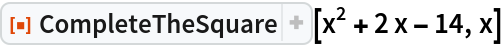Out=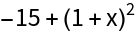Complete the square in x of a quadratic polynomial in x and y:

 In:=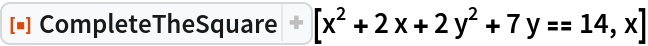Out=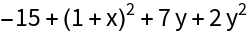Complete the square in both x and y:

 In:=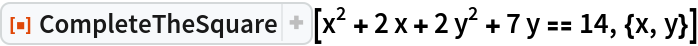Out=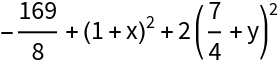Symbolic coefficients are allowed:

 In:=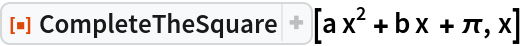Out=Complete the square symbolically for a trivariate quadratic polynomial:

 In:=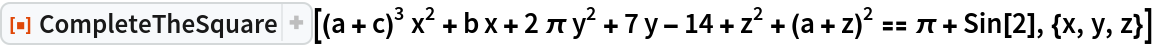Out=### Possible Issues (3)

If the expression is not a polynomial, an error message is returned:

 In:=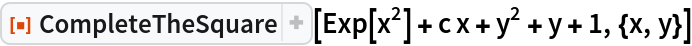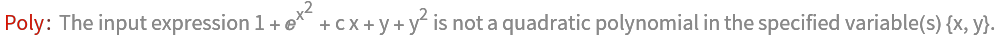If the expression is not a polynomial of degree 2, an error message is returned:

 In:=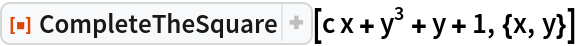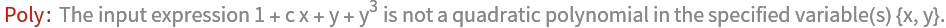If the polynomial contains mixed terms, an error message is returned:

 In:=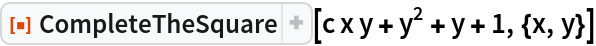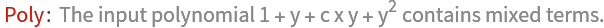## Publisher

Dennis M Schneider

## Version History

• 1.0.0 – 09 August 2019